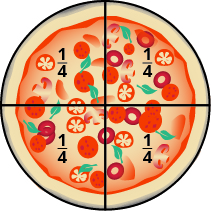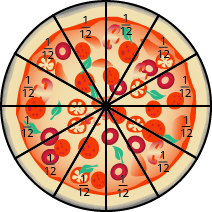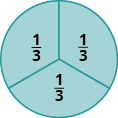## Convert Between Types of Fractions

### Learning Outcomes

• Identify different types of fractions and convert between them

Andy and Bobby love pizza. On Tuesday night, Andy and Bobby share a pizza with their parents, Fred and Christy, with each person getting an equal amount of the whole pizza. How much of the pizza does each person get? There is one whole pizza, divided evenly into four equal parts. Each person has one of the four equal parts, so each has $\Large{\frac{1}{4}}$ of the pizza.On Wednesday, the family invites some friends over for a pizza dinner. There are a total of $12$ people. If they share the pizza equally, each person would get $\Large{\frac{1}{12}}$ of the pizza.### Fractions

A fraction is written $\Large{\frac{a}{b}}$, where $a$ and $b$ are integers and $b\ne 0$. In a fraction, $a$ is called the numerator and $b$ is called the denominator.

A fraction is a way to represent parts of a whole. The denominator $b$ represents the number of equal parts the whole has been divided into, and the numerator $a$ represents how many parts are included. The denominator, $b$, cannot equal zero because division by zero is undefined.

In the image below, the circle has been divided into three parts of equal size. Each part represents $\Large{\frac{1}{3}}$ of the circle. This type of model is called a fraction circle. Other shapes, such as rectangles, can also be used to model fractions.What does the fraction $\Large{\frac{2}{3}}$ represent? The fraction $\Large{\frac{2}{3}}$ means two of three equal parts.Watch the following video to see more examples of how to write fractions given a model.

## Mixed Numbers and Improper Fractions

What would happen if you have eight equal fifth pieces. You can use five of them to make one whole, but you’ll have three fifths left over. Let us use fraction notation to show what happened. You had eight pieces, each of them one fifth, ${\Large\frac{1}{5}}$, so altogether you had eight fifths, which we can write as ${\Large\frac{8}{5}}$. The fraction ${\Large\frac{8}{5}}$ is one whole, $1$, plus three fifths, ${\Large\frac{3}{5}}$, or $1{\Large\frac{3}{5}}$, which is read as one and three-fifths.

The number $1{\Large\frac{3}{5}}$ is called a mixed number.

### Mixed Numbers

A mixed number consists of a whole number $a$ and a fraction ${\Large\frac{b}{c}}$ where $c\ne 0$. It is written as follows.

$a{\Large\frac{b}{c}}\text{, }c\ne 0$

The number ${\Large\frac{8}{5}}$ is called an improper fraction.

### Proper and Improper Fractions

The fraction ${\Large\frac{a}{b}}$ is a proper fraction if $a<b$ and an improper fraction if $a\ge b$.

Fractions such as ${\Large\frac{5}{4}},{\Large\frac{3}{2}},{\Large\frac{5}{5}}$, and ${\Large\frac{7}{3}}$ are called improper fractions. In an improper fraction, the numerator is greater than or equal to the denominator, so its value is greater than or equal to one. When a fraction has a numerator that is smaller than the denominator, it is called a proper fraction, and its value is less than one. Fractions such as ${\Large\frac{1}{2}},{\Large\frac{3}{7}}$, and ${\Large\frac{11}{18}}$ are proper fractions.

### Example

Draw a figure to model ${\Large\frac{11}{8}}$.

### Try it

Draw a figure to model ${\Large\frac{7}{6}}$

Draw a figure to model ${\Large\frac{6}{5}}$

### Example

Name the improper fraction modeled. Then write the improper fraction as a mixed number.### Example

Use a model to rewrite the improper fraction ${\Large\frac{11}{6}}$ as a mixed number.

### Try it

In the next video we show another way to draw a model that represents a fraction.  You will see example of both proper and improper fractions shown.

### Example

Use a model to rewrite the mixed number $1{\Large\frac{4}{5}}$ as an improper fraction.

### Try it

There is another method to turning a mixed number into an improper fraction — it’s just a shortcut to what you’ve been practicing above.

### Mixed Numbers to Improper Fractions

1. Multiply the whole number by the denominator
2. Add that value to the numerator (this becomes the numerator of the improper fraction)
3. Place the denominator of the mixed number in the denominator of the improper fraction

### EXAMPLE

Convert $5\frac{2}{3}$ into an improper fraction using the shortcut

 1. Multiply the whole number by the denomimator $5\cdot{3}=15$ 2. Add that value to the numerator $15+2=17$ new numerator for improper fraction $\Large\frac{17}{?}$ 3. Place the denominator of the mixed number in the denominator of the improper fraction $\Large\frac{17}{3}$

## Contribute!

Did you have an idea for improving this content? We’d love your input.